# Scatter plot with marginal histograms in ggplot2

Dean Attali

## Adding marginal histograms with `ggExtra`

The `ggMarginal` function of the `ggExtra` package allows adding marginal histograms to an existing scatter plot. For that purpose you will need to store the scatter plot made with ggplot2 inside a variable and pass it to `ggMarginal`, specifying `type = "histogram"`.

``````# install.packages("ggplot2")
# install.packages("ggExtra")
library(ggplot2)
library(ggExtra)

# Save the scatter plot in a variable
p <- ggplot(cars, aes(x = speed, y = dist)) +
geom_point()

# Plot the scatter plot with marginal histograms
ggMarginal(p, type = "histogram")``````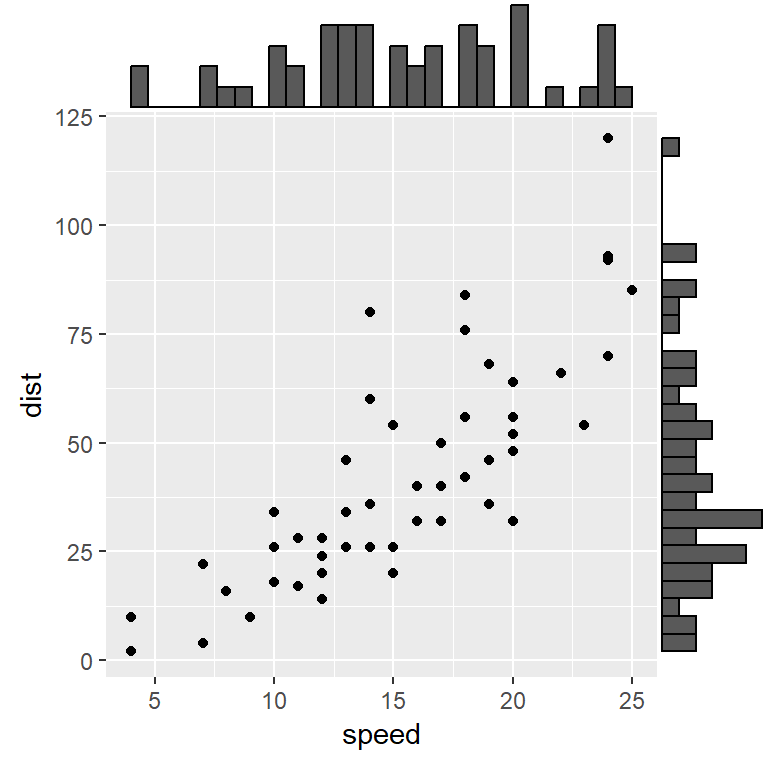Histogram only on the horizontal axis

The `margins` argument can be used to set only one of the marginal histograms, as the X-axis histogram.

``````# install.packages("ggplot2")
# install.packages("ggExtra")
library(ggplot2)
library(ggExtra)

# Save the scatter plot in a variable
p <- ggplot(cars, aes(x = speed, y = dist)) +
geom_point()

# Horizontal marginal histogram
ggMarginal(p, type = "histogram",
margins = "x")``````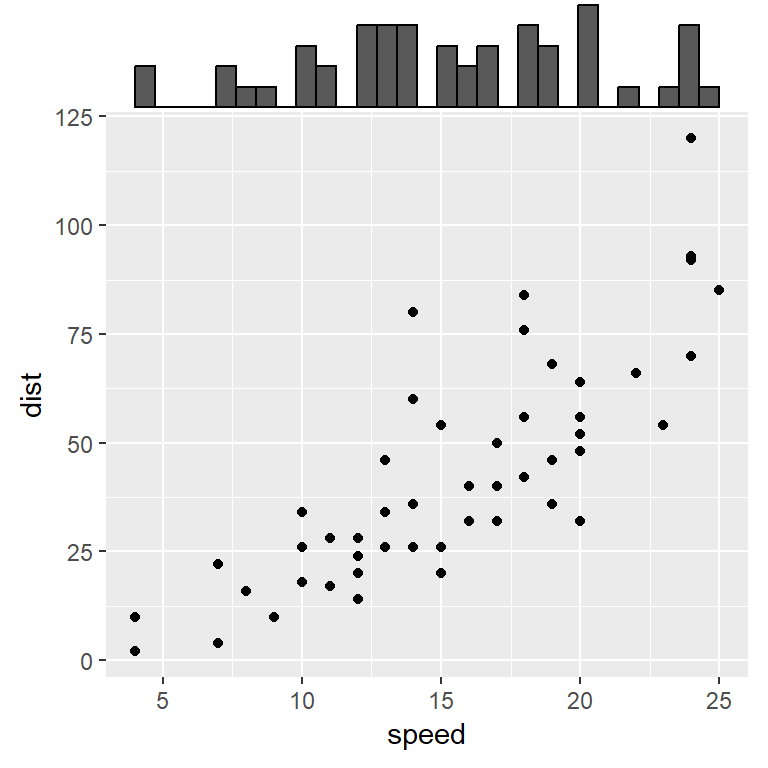Histogram only on the vertical axis

If you prefer showing only the Y-axis histogram set `margins = "y"`.

``````# install.packages("ggplot2")
# install.packages("ggExtra")
library(ggplot2)
library(ggExtra)

# Save the scatter plot in a variable
p <- ggplot(cars, aes(x = speed, y = dist)) +
geom_point()

# Vertical marginal histogram
ggMarginal(p, type = "histogram",
margins = "y")``````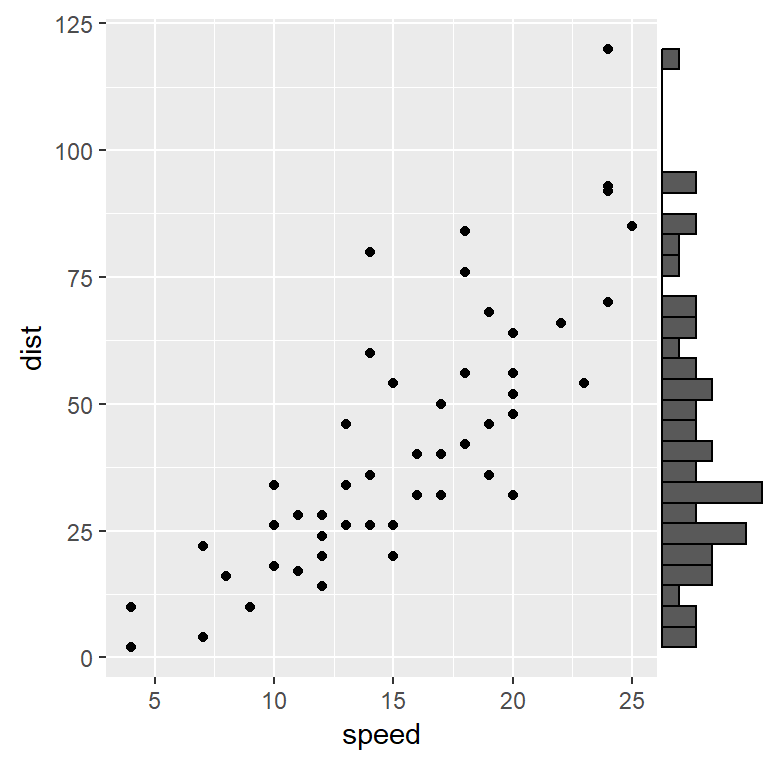Relative size

The `size` argument modifies the relative size between the histogram and the scatter plot. The default value is 5 (scatter plot 5 times bigger than the histograms).

``````# install.packages("ggplot2")
# install.packages("ggExtra")
library(ggplot2)
library(ggExtra)

# Save the scatter plot in a variable
p <- ggplot(cars, aes(x = speed, y = dist)) +
geom_point()

# Changing the relative size
ggMarginal(p, type = "histogram",
size = 3)``````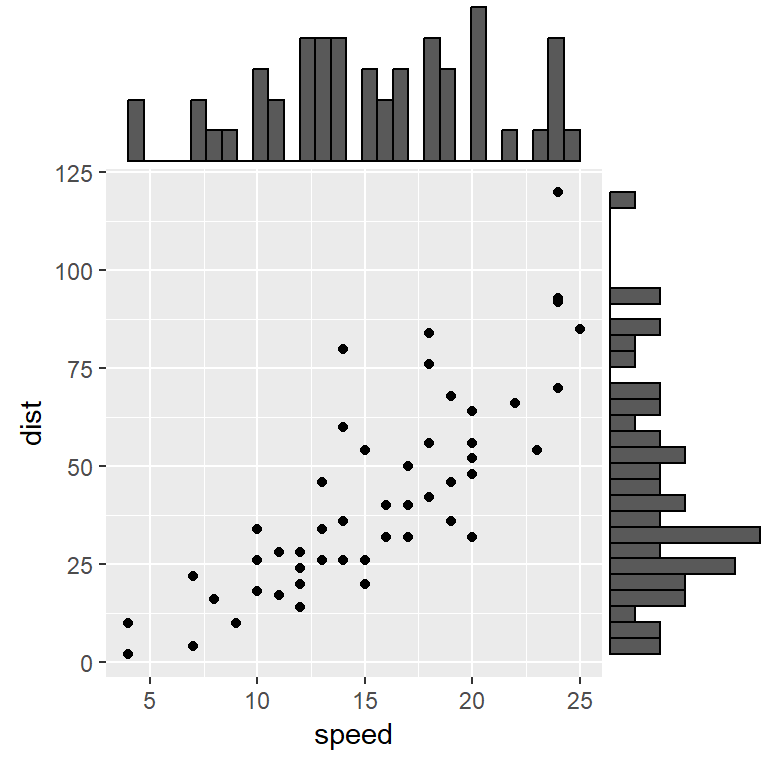Arguments to be passed to `geom_boxplot`

You can pass the arguments of `geom_boxplot` to the function. If you want to set arguments for each histogram pass lists to `xparams` and `yparams`.

``````# install.packages("ggplot2")
# install.packages("ggExtra")
library(ggplot2)
library(ggExtra)

# Save the scatter plot in a variable
p <- ggplot(cars, aes(x = speed, y = dist)) +
geom_point()

# Bin width customization
ggMarginal(p, type = "histogram",
binwidth = 4)``````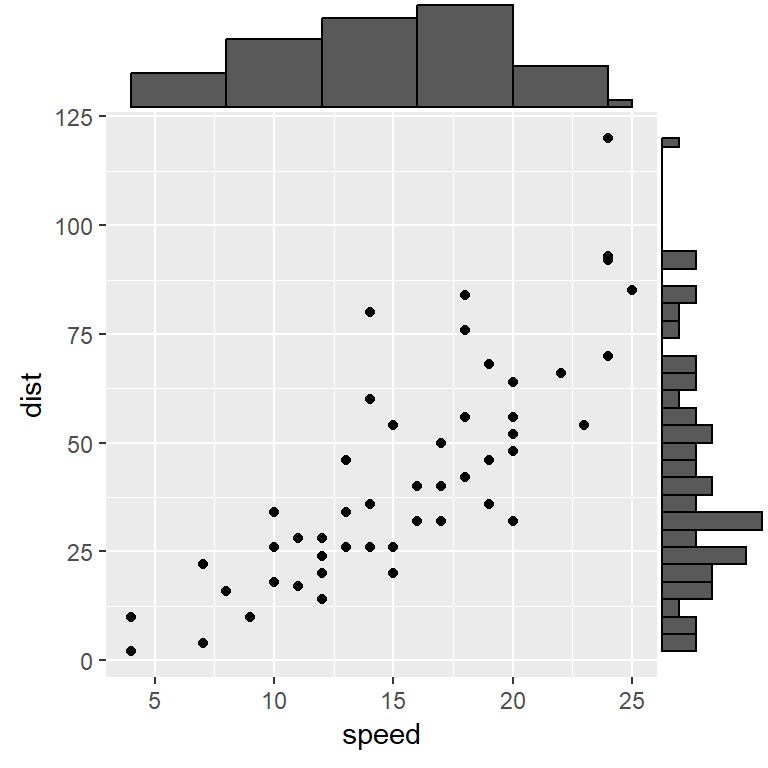Histogram with density lines

If you want to overlay density lines to the histograms set `type = "densigram"`.

``````# install.packages("ggplot2")
# install.packages("ggExtra")
library(ggplot2)
library(ggExtra)

# Save the scatter plot in a variable
p <- ggplot(cars, aes(x = speed, y = dist)) +
geom_point()

# Densigram
ggMarginal(p, type = "densigram")``````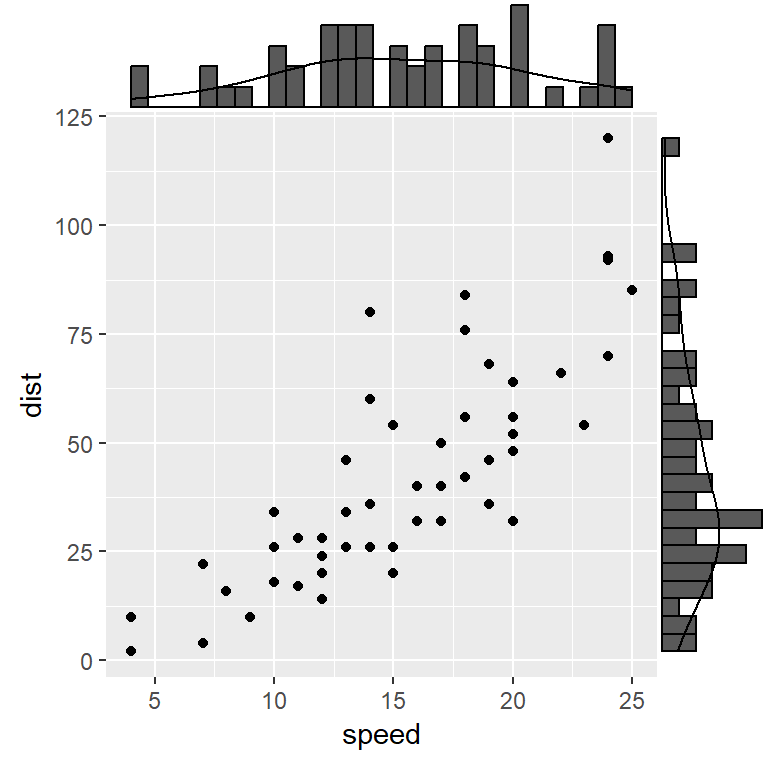## Color customization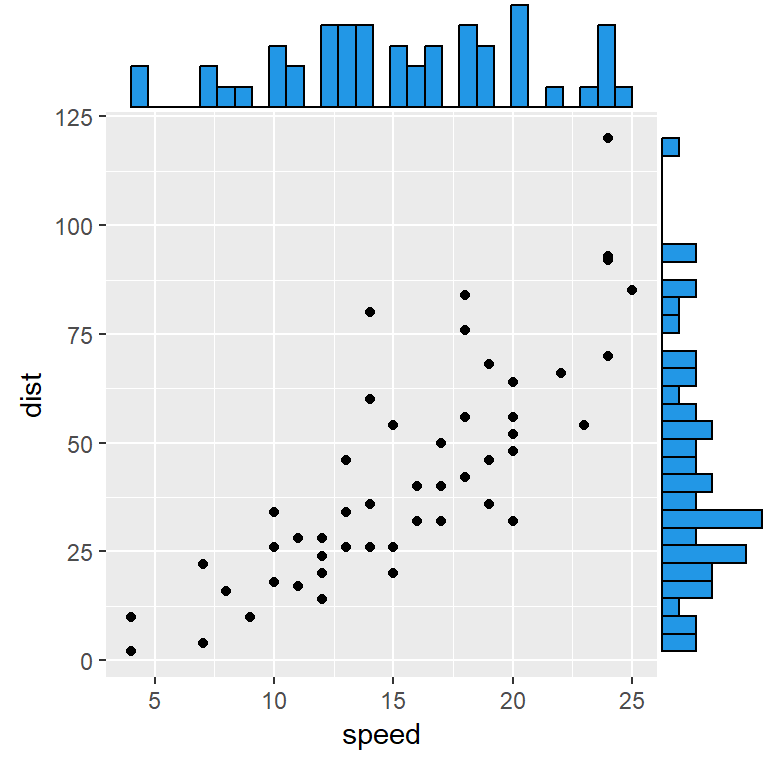Fill color

Change the fill color of the histograms with `fill`.

``````# install.packages("ggplot2")
# install.packages("ggExtra")
library(ggplot2)
library(ggExtra)

# Save the scatter plot in a variable
p <- ggplot(cars, aes(x = speed, y = dist)) +
geom_point()

# Changing the fill color
ggMarginal(p, type = "histogram",
fill = 4)``````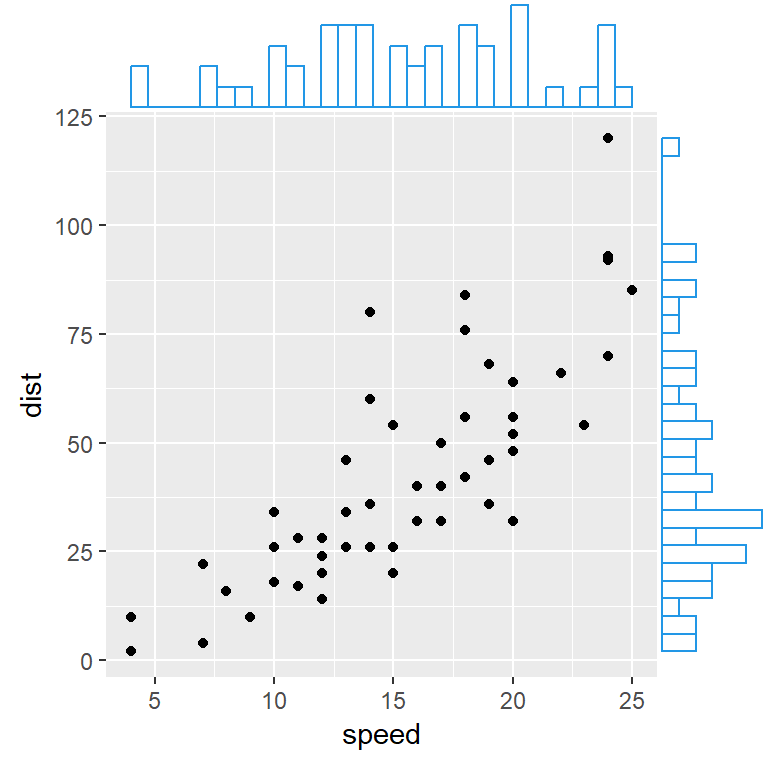Border color

You can also customize the border color with `col` argument.

``````# install.packages("ggplot2")
# install.packages("ggExtra")
library(ggplot2)
library(ggExtra)

# Save the scatter plot in a variable
p <- ggplot(cars, aes(x = speed, y = dist)) +
geom_point()

# Fill and border color of the marginal histograms
ggMarginal(p, type = "histogram",
fill = "white",
col = 4)``````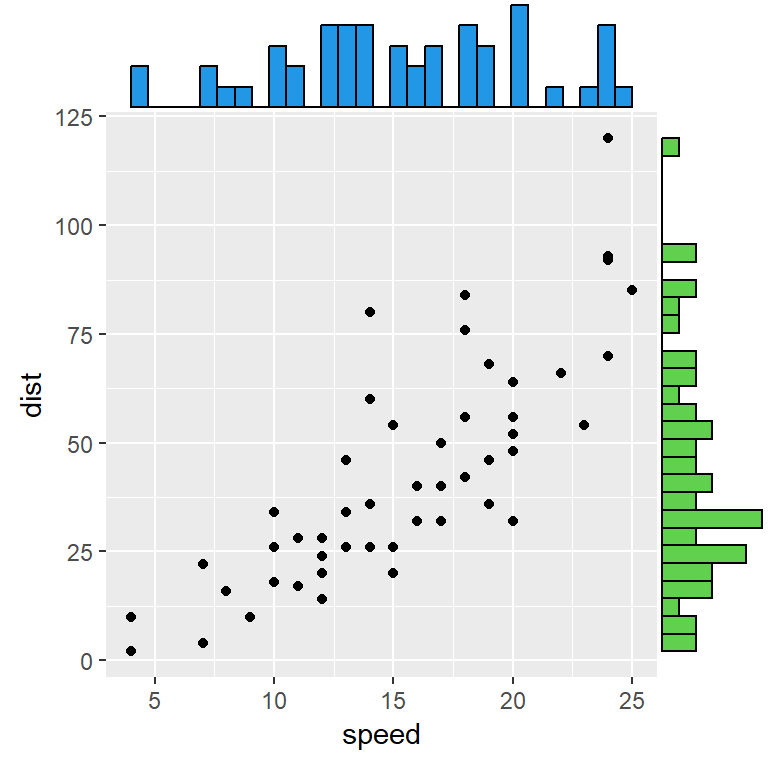Arguments for each histogram

If you want more customization, pass a list or arguments for each axis with `xparams` and `yparams`.

``````# install.packages("ggplot2")
# install.packages("ggExtra")
library(ggplot2)
library(ggExtra)

# Save the scatter plot in a variable
p <- ggplot(cars, aes(x = speed, y = dist)) +
geom_point()

# Arguments for each marginal histogram
ggMarginal(p, type = "histogram",
xparams = list(fill = 4),
yparams = list(fill = 3))``````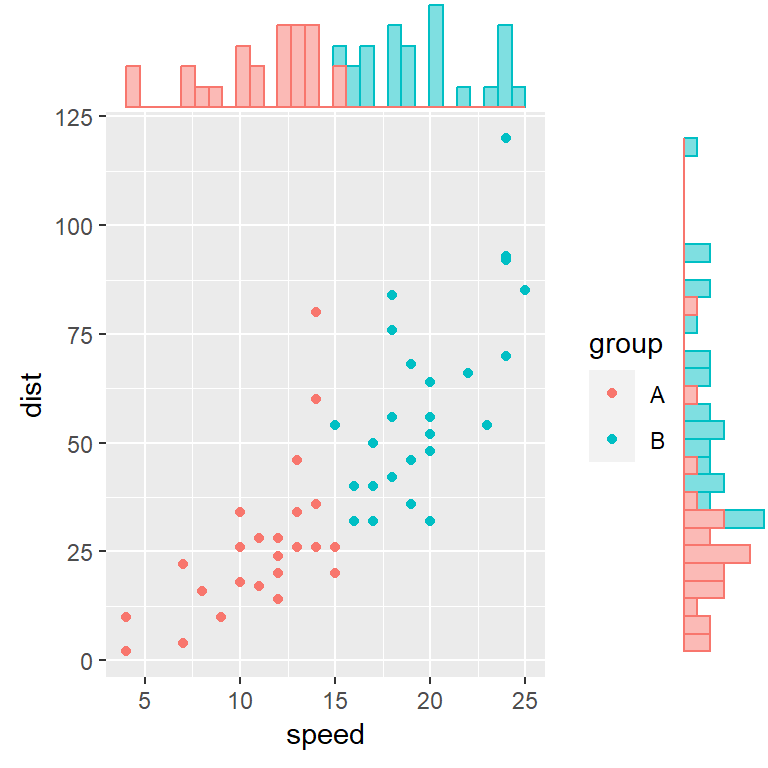Histograms by group

Finally, if your data set contains a grouping variable you can fill the marginal histograms by group.

``````# install.packages("ggplot2")
# install.packages("ggExtra")
library(ggplot2)
library(ggExtra)

# Sample grouping variable
cars\$group <- c(rep("A", 25), rep("B", 25))

# Save the scatter plot in a variable
p <- ggplot(cars, aes(x = speed, y = dist, color = group)) +
geom_point()

# Marginal histograms by group
ggMarginal(p, type = "histogram",
groupColour = TRUE,
groupFill = TRUE)``````